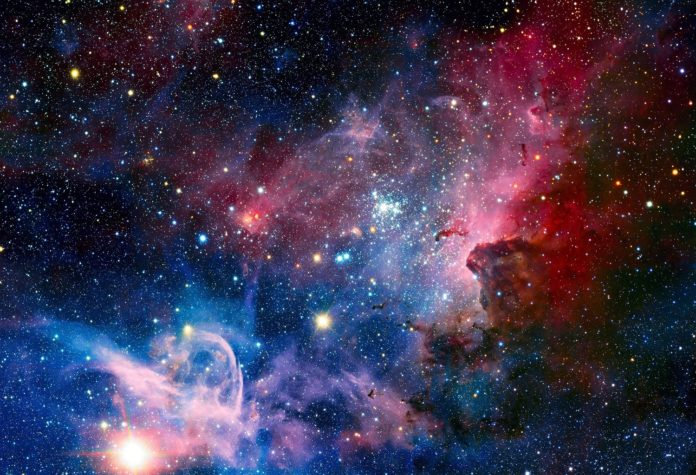# 3135

0
116Researchers from South Ural State University Igor Klebanov and Sergey Ivanov are the first ones in the world to find symmetries which are admitted by the system of equations on self-gravitating polytrophic gas. Using the method of group analysis, they are studying physical processes in the Universe.

The method of group analysis, which is used by the researchers, was thought up by a Norwegian mathematician Sophus Lie in the 19th century. The gist of the method is in searching for symmetry. Finding these transformations which do not change the form of the equations, we can find out much about possible solutions.

“Group analysis is a mathematical method which makes it possible to study systems of differential equations which model some kind of process and see the solutions that we cannot see otherwise,” says Igor Klebanov.

Researchers have previously completed a group analysis and found new exact solutions for the model of Newtonian Cosmology. The obtained model is a system of equations of the self-gravitating gas dynamics, and when this gas moves, its gravitational field must be taken into account. This model can be used in the theory of stars forming from interstellar gas.

SUSU researchers are the first in the world to find symmetries which allow for a system of equations for self-gravitating polytrophic gas, which means that researchers should continue searching for new analytical solutions of this model, one of which has already been found. This solution opens up huge possibilities for astrophysicists who need to verify the results.

An article on the results of this research was published in the Communications in Nonlinear Science and Numerical Simulation journal, listed in the Web of Science Top 10%.

Photo by PressFoto